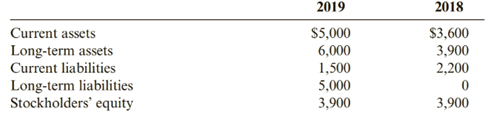Chapter 12, Problem 62BE### Cornerstones of Financial Accounti...

4th Edition
Jay Rich + 1 other
ISBN: 9781337690881

#### Solutions

Chapter
Section### Cornerstones of Financial Accounti...

4th Edition
Jay Rich + 1 other
ISBN: 9781337690881
Textbook Problem
5 views

# Debt Management and Short-Term Liquidity RatiosMagellan Company is an international travel agency providing travel planning services to customers in over 20 Countries. Recently, the travel industry has been experiencing volatility as a result of increases in oil prices. Magellan’s investors have been following its financial information closely to determine its ability to continue as a going concern, Its investors have used the following information to determine financial ratios:Required:Between 20l8 and 20l9, indicate whether Magellan’s debt to equity ratio increased or decreased. Also, indicate whether Magellan’s current ratio increased or decreased. Interpret these ratios.

To determine

Introduction:

Debt-equity ratio is calculated by dividing the total debt of the company to the shareholders fund. It is used to compute the financial leverage of a company.

Current ratio is the ratio of current assets to current liabilities.

To find:

The debt-equity ratio and current ratio of Figgins’ for the year 2018 and 2019 and comparing the results.

Explanation
• Debt-equity ratio

Debt-equity ratio=Total liabilitiesTotal equity

Total liabilities for 2018 = Long-term liabilities + Current liabilities

= 0 + $2,200 =$2,200

Total liabilities for 2019 = $5,000 +$ 1,500

= $6,500 Debt-equity ratio for 2018=$2,200$3,900 = 0.56: 1 Debt-equity ratio for 2019=$6,500\$3,900

= 1

### Still sussing out bartleby?

Check out a sample textbook solution.

See a sample solution

#### The Solution to Your Study Problems

Bartleby provides explanations to thousands of textbook problems written by our experts, many with advanced degrees!

Get Started

#### Find more solutions based on key concepts